Comparison
Comparison close
Results

CCM.P-K2, EURAMET.M.P-K4.2010 and APMP.M.P-K9

MEASURAND                     Effective area of a piston-cylinder assembly

NOMINAL VALUE              335.7 mm2

NOMINAL PRESSURE        10 kPa

CCM.P-K2

Degrees of equivalence represented by Di = (xxR) and its expanded uncertainty Ui at a 95 % level of confidence, both expressed in mm2.

EURAMET.M.P-K4.2010 and APMP.M.P-K9

Degrees of equivalence represented by Di = (xI - xR) and its expanded uncertainty Ui at a 95 % level of confidence, both expressed in Pa.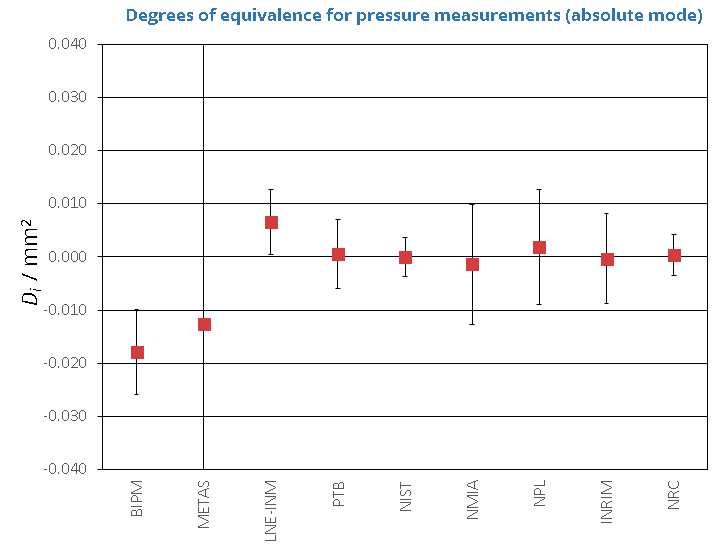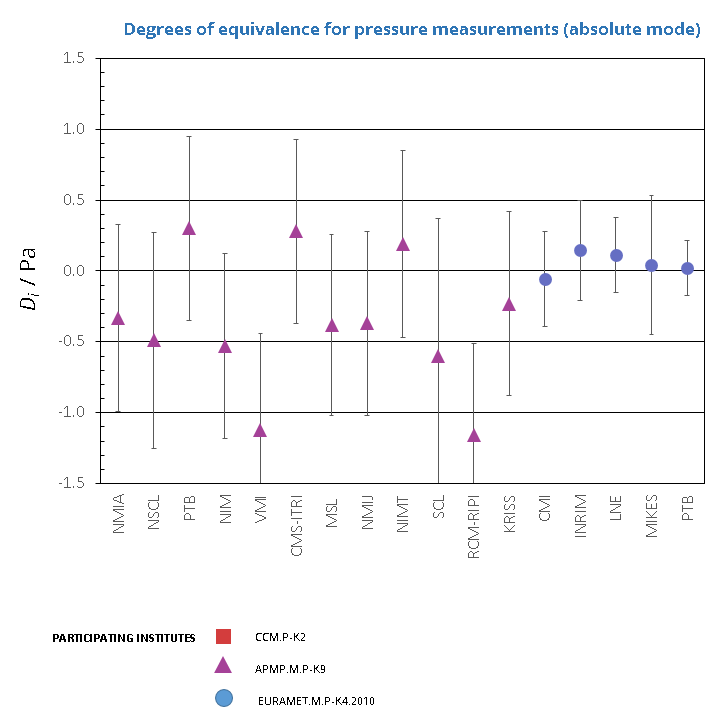CCM.P-K2 and EURAMET.M.P-K8

MEASURAND                     Effective area of a piston-cylinder assembly

NOMINAL VALUE              335.7 mm2

NOMINAL PRESSURE       20 kPa

Degrees of equivalence represented by Di = (xxR) and its expanded uncertainty Ui at a 95 % level of confidence, both expressed in mm2.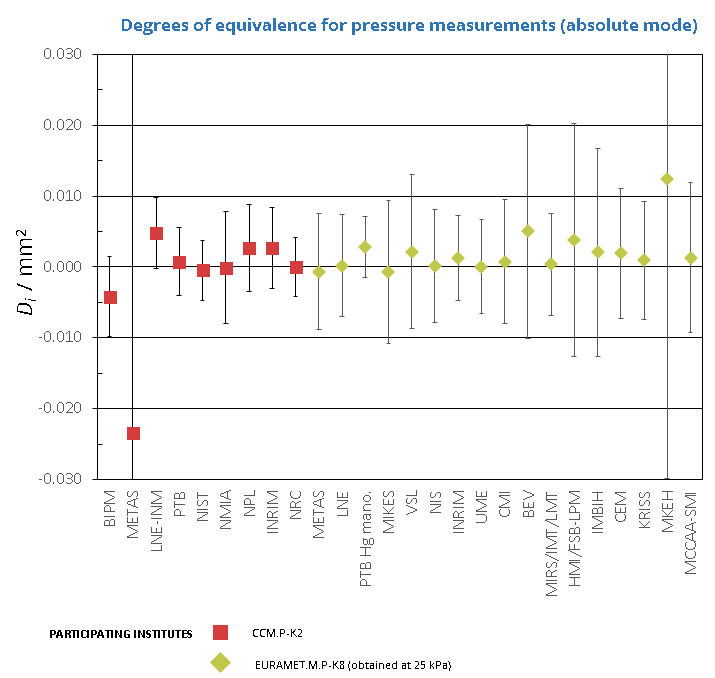CCM.P-K2 and APMP.M.P-K9

MEASURAND                     Effective area of a piston-cylinder assembly

NOMINAL VALUE              335.7 mm2

NOMINAL PRESSURE       30 kPa

CCM.P-K2

Degrees of equivalence represented by Di = (xxR) and its expanded uncertainty Ui at a 95 % level of confidence, both expressed in mm2.

APMP.M.P-K9

Degrees of equivalence represented by Di = (xI - xR) and its expanded uncertainty Ui at a 95 % level of confidence, both expressed in Pa.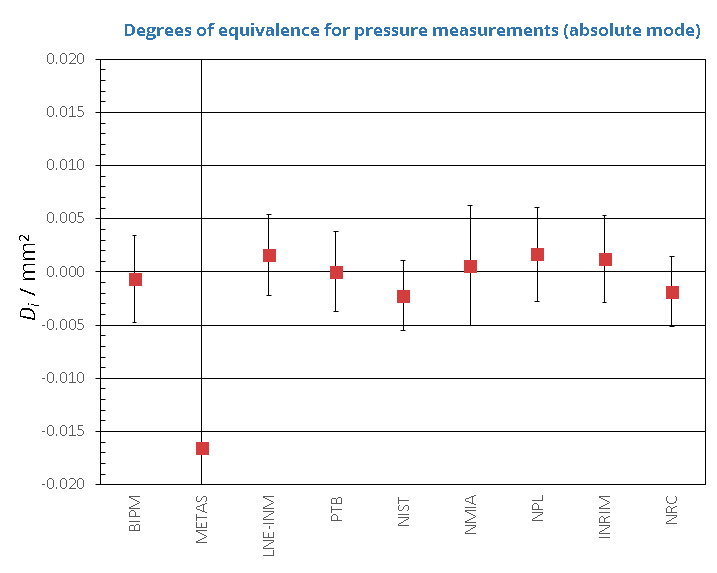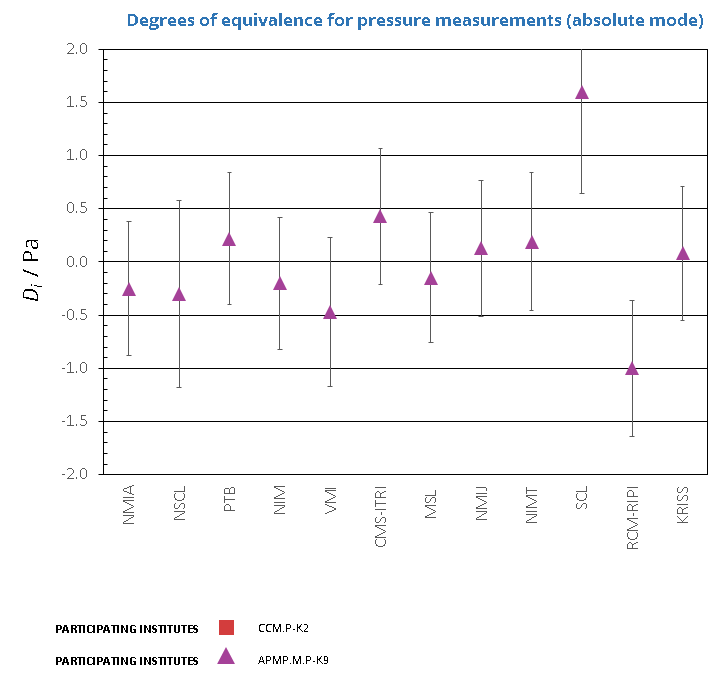CCM.P-K2

MEASURAND                     Effective area of a piston-cylinder assembly

NOMINAL VALUE              335.7 mm2

NOMINAL PRESSURE       40 kPa

Degrees of equivalence represented by Di = (xxR) and its expanded uncertainty Ui at a 95 % level of confidence, both expressed in mm2.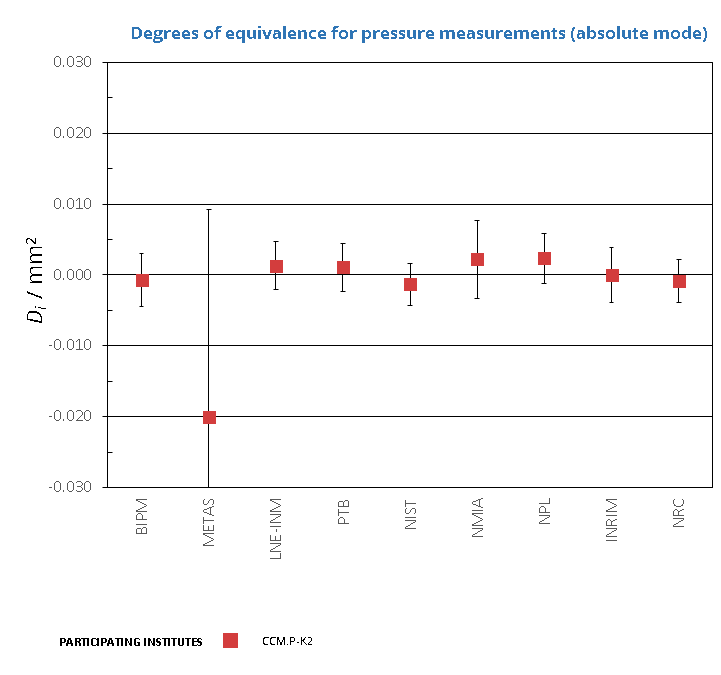CCM.P-K2, EURAMET.M.P-K8 and APMP.M.P-K9

MEASURAND                     Effective area of a piston-cylinder assembly

NOMINAL VALUE              335.7 mm2

NOMINAL PRESSURE       50 kPa

CCM.P-K2 and EURAMET.M.P-K8

Degrees of equivalence represented by Di = (xxR) and its expanded uncertainty Ui at a 95 % level of confidence, both expressed in mm2.

APMP.M.P-K9

Degrees of equivalence represented by Di = (xI - xR) and its expanded uncertainty Ui at a 95 % level of confidence, both expressed in Pa.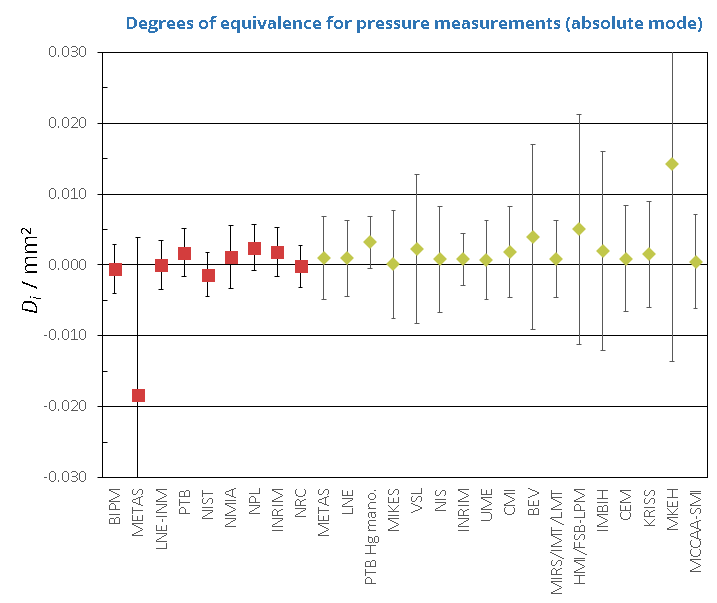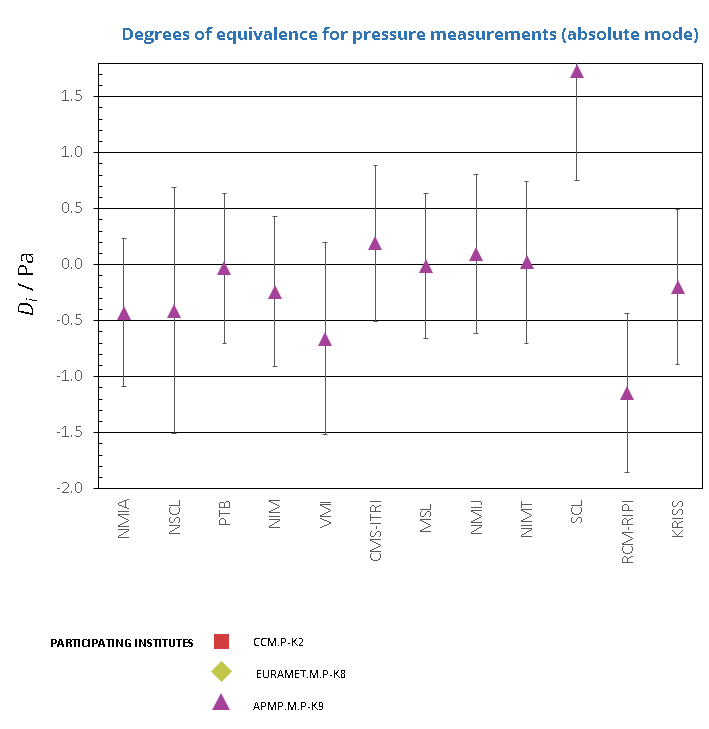CCM.P-K2

MEASURAND                     Effective area of a piston-cylinder assembly

NOMINAL VALUE              335.7 mm2

NOMINAL PRESSURE       60 kPa

Degrees of equivalence represented by Di = (xxR) and its expanded uncertainty Ui at a 95 % level of confidence, both expressed in mm2.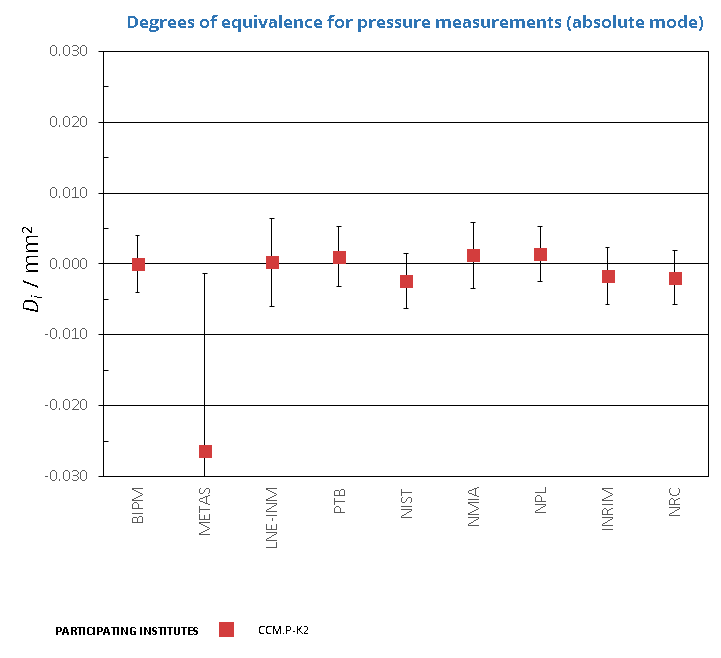CCM.P-K2, EURAMET.M.P-K8 and APMP.M.P-K9

MEASURAND                     Effective area of a piston-cylinder assembly

NOMINAL VALUE              335.7 mm2

NOMINAL PRESSURE       70 kPa

CCM.P-K2 and EURAMET.M.P-K8

Degrees of equivalence represented by Di = (xxR) and its expanded uncertainty Ui at a 95 % level of confidence, both expressed in mm2.

APMP.M.P-K9

Degrees of equivalence represented by Di = (xI - xR) and its expanded uncertainty Ui at a 95 % level of confidence, both expressed in Pa.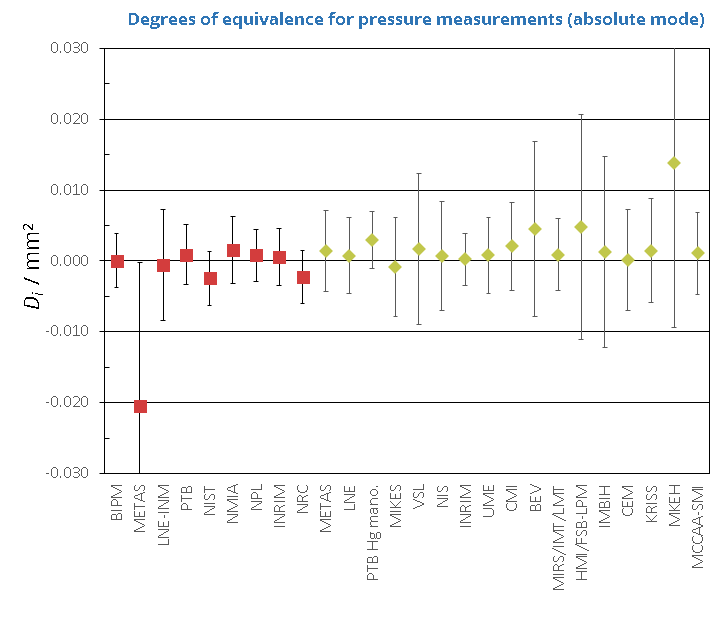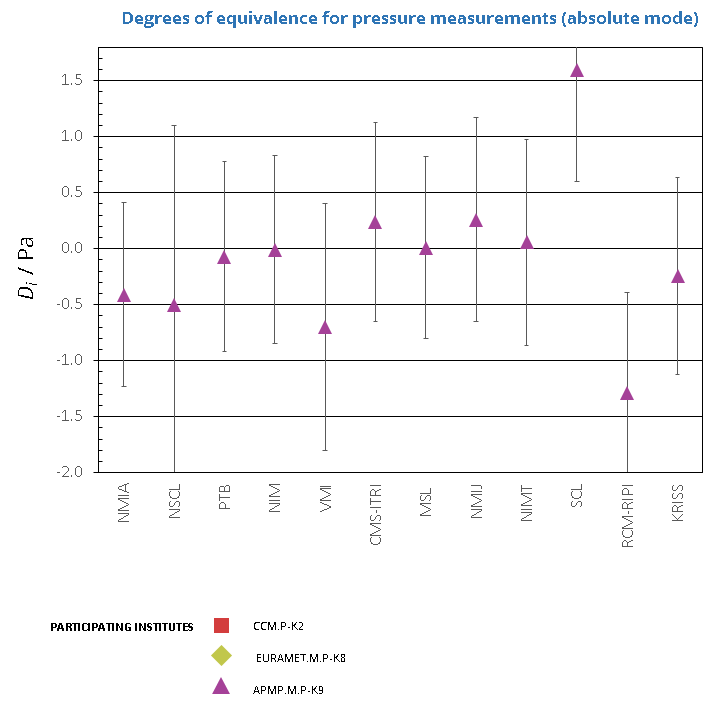CCM.P-K2

MEASURAND                     Effective area of a piston-cylinder assembly

NOMINAL VALUE              335.7 mm2

NOMINAL PRESSURE       80 kPa

Degrees of equivalence represented by Di = (xxR) and its expanded uncertainty Ui at a 95 % level of confidence, both expressed in mm2.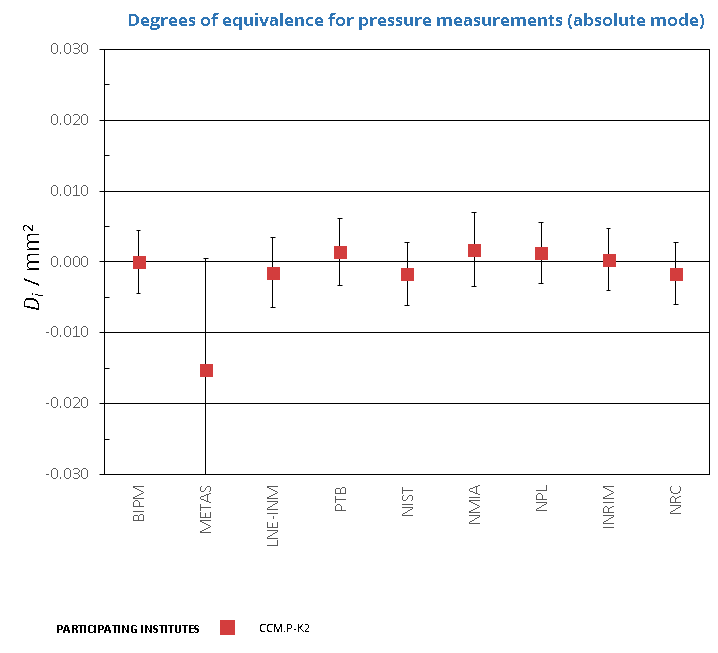CCM.P-K2 and APMP.M.P-K9

MEASURAND                     Effective area of a piston-cylinder assembly

NOMINAL VALUE              335.7 mm2

NOMINAL PRESSURE       90 kPa

CCM.P-K2

Degrees of equivalence represented by Di = (xxR) and its expanded uncertainty Ui at a 95 % level of confidence, both expressed in mm2.

APMP.M.P-K9

Degrees of equivalence represented by Di = (xI - xR) and its expanded uncertainty Ui at a 95 % level of confidence, both expressed in Pa.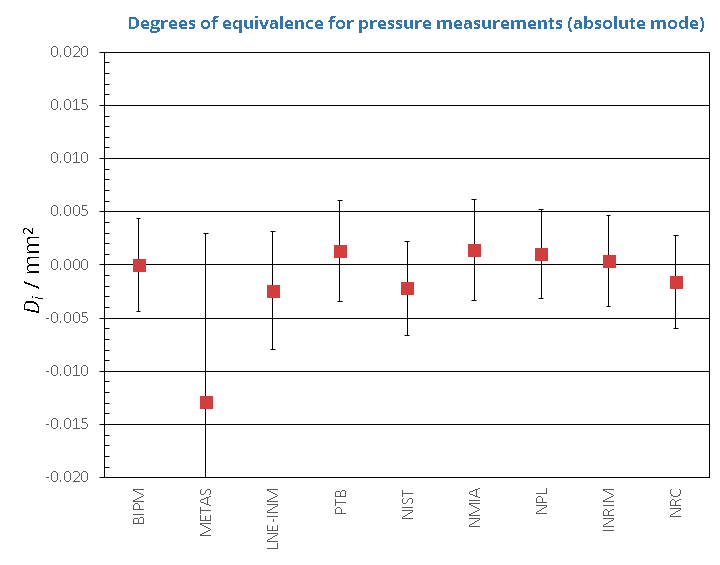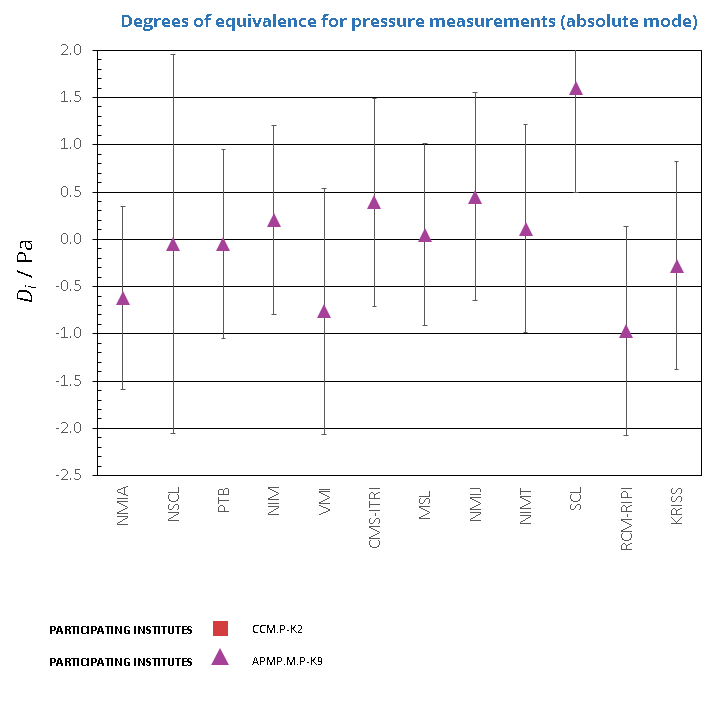CCM.P-K2, EURAMET.M.P-K8 and APMP.M.P-K9

MEASURAND                     Effective area of a piston-cylinder assembly

NOMINAL VALUE              335.7 mm2

NOMINAL PRESSURE       100 kPa

CCM.P-K2 and EURAMET.M.P-K8

Degrees of equivalence represented by Di = (xxR) and its expanded uncertainty Ui at a 95 % level of confidence, both expressed in mm2.

APMP.M.P-K9

Degrees of equivalence represented by Di = (xI - xR) and its expanded uncertainty Ui at a 95 % level of confidence, both expressed in Pa.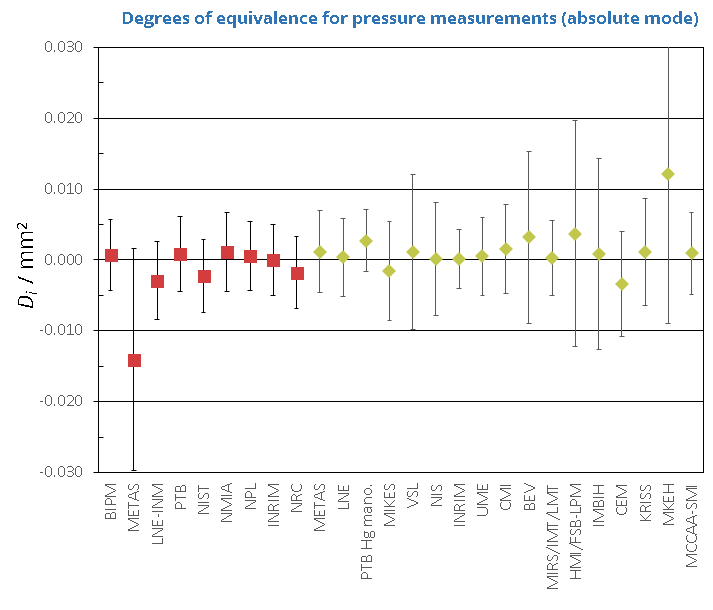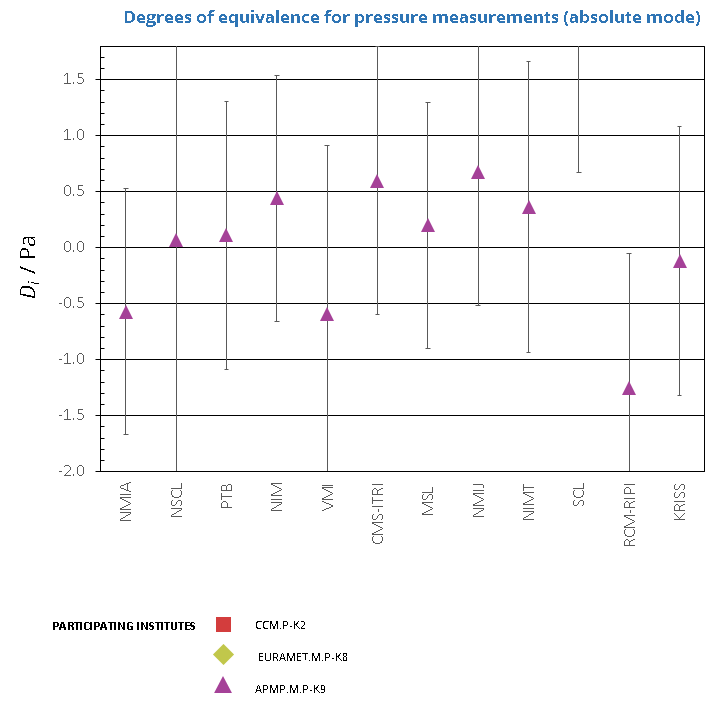CCM.P-K2 and APMP.M.P-K9

MEASURAND                     Effective area of a piston-cylinder assembly

NOMINAL VALUE              335.7 mm2

NOMINAL PRESSURE       110 kPa

CCM.P-K2

Degrees of equivalence represented by Di = (xxR) and its expanded uncertainty Ui at a 95 % level of confidence, both expressed in mm2.

APMP.M.P-K9

Degrees of equivalence represented by Di = (xI - xR) and its expanded uncertainty Ui at a 95 % level of confidence, both expressed in Pa.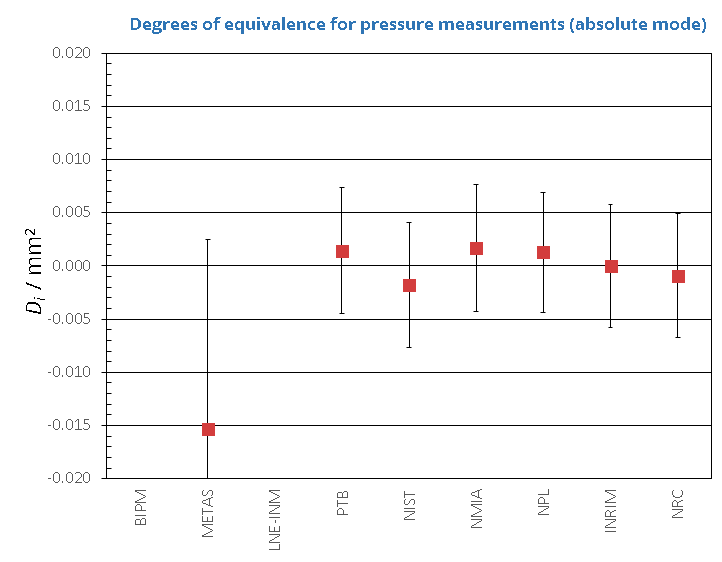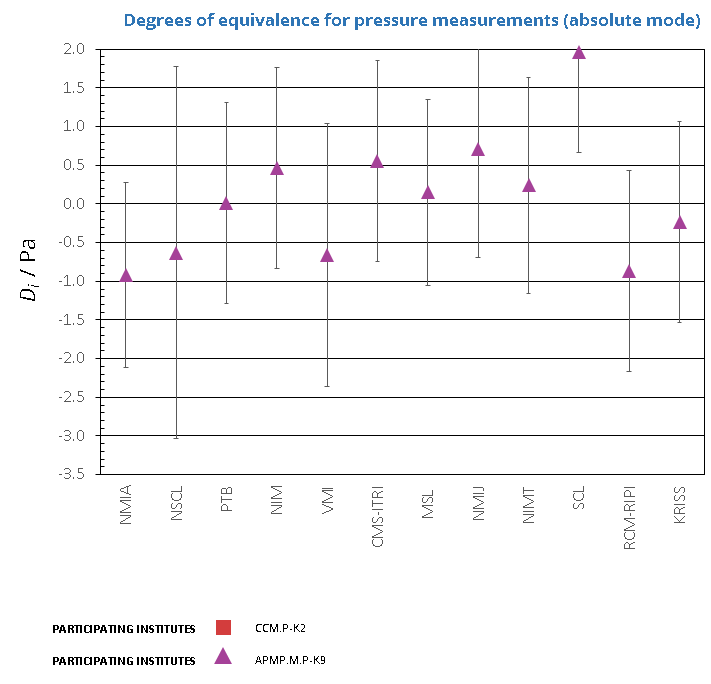CCM.P-K2

MEASURAND                     Effective area of a piston-cylinder assembly

NOMINAL VALUE              335.7 mm2

NOMINAL PRESSURE       120 kPa

Degrees of equivalence represented by Di = (xxR) and its expanded uncertainty Ui at a 95 % level of confidence, both expressed in mm2.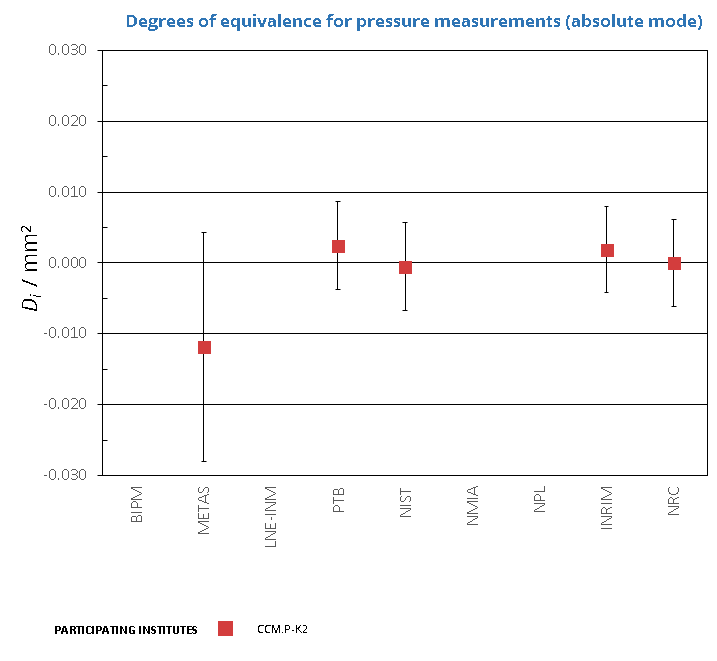Comparison
Comparison close
Results

CCM.P-K2, EURAMET.M.P-K4.2010 and APMP.M.P-K9

MEASURAND                     Effective area of a piston-cylinder assembly

NOMINAL VALUE              335.7 mm2

NOMINAL PRESSURE        10 kPa

CCM.P-K2

Degrees of equivalence represented by Di = (xxR) and its expanded uncertainty Ui at a 95 % level of confidence, both expressed in mm2.

EURAMET.M.P-K4.2010 and APMP.M.P-K9

Degrees of equivalence represented by Di = (xI - xR) and its expanded uncertainty Ui at a 95 % level of confidence, both expressed in Pa.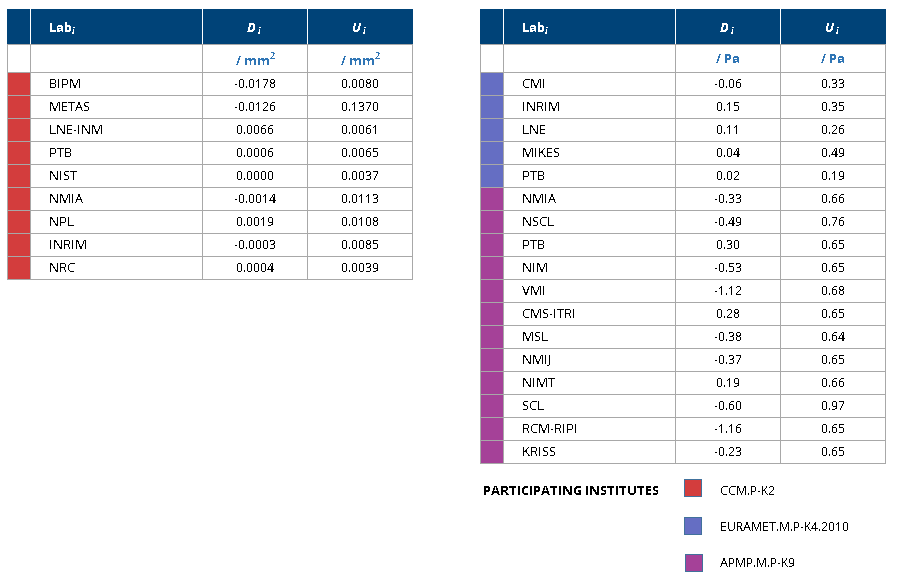CCM.P-K2 and EURAMET.M.P-K8

MEASURAND                     Effective area of a piston-cylinder assembly

NOMINAL VALUE              335.7 mm2

NOMINAL PRESSURE       20 kPa

Degrees of equivalence represented by Di = (xxR) and its expanded uncertainty Ui at a 95 % level of confidence, both expressed in mm2.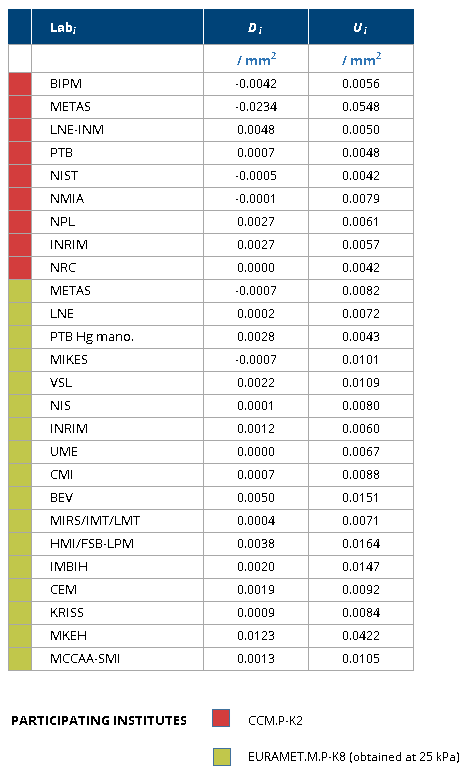CCM.P-K2 and APMP.M.P-K9

MEASURAND                     Effective area of a piston-cylinder assembly

NOMINAL VALUE              335.7 mm2

NOMINAL PRESSURE       30 kPa

CCM.P-K2

Degrees of equivalence represented by Di = (xxR) and its expanded uncertainty Ui at a 95 % level of confidence, both expressed in mm2.

APMP.M.P-K9

Degrees of equivalence represented by Di = (xI - xR) and its expanded uncertainty Ui at a 95 % level of confidence, both expressed in Pa.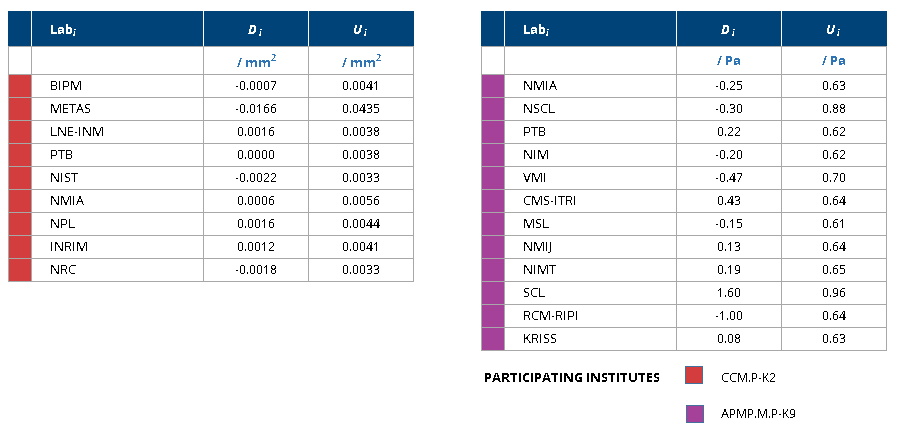CCM.P-K2

MEASURAND                     Effective area of a piston-cylinder assembly

NOMINAL VALUE              335.7 mm2

NOMINAL PRESSURE       40 kPa

Degrees of equivalence represented by Di = (xxR) and its expanded uncertainty Ui at a 95 % level of confidence, both expressed in mm2.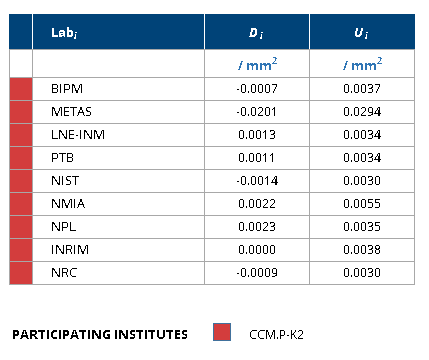CCM.P-K2, EURAMET.M.P-K8 and APMP.M.P-K9

MEASURAND                     Effective area of a piston-cylinder assembly

NOMINAL VALUE              335.7 mm2

NOMINAL PRESSURE       50 kPa

CCM.P-K2 and EURAMET.M.P-K8

Degrees of equivalence represented by Di = (xxR) and its expanded uncertainty Ui at a 95 % level of confidence, both expressed in mm2.

APMP.M.P-K9

Degrees of equivalence represented by Di = (xI - xR) and its expanded uncertainty Ui at a 95 % level of confidence, both expressed in Pa.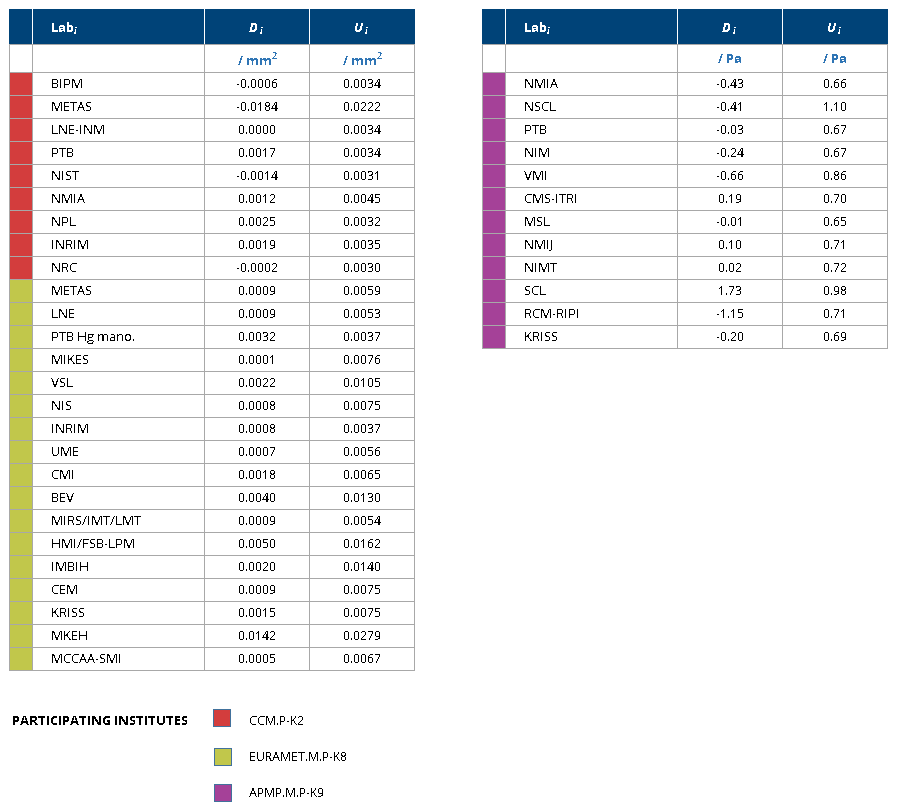CCM.P-K2

MEASURAND                     Effective area of a piston-cylinder assembly

NOMINAL VALUE              335.7 mm2

NOMINAL PRESSURE       60 kPa

Degrees of equivalence represented by Di = (xxR) and its expanded uncertainty Ui at a 95 % level of confidence, both expressed in mm2.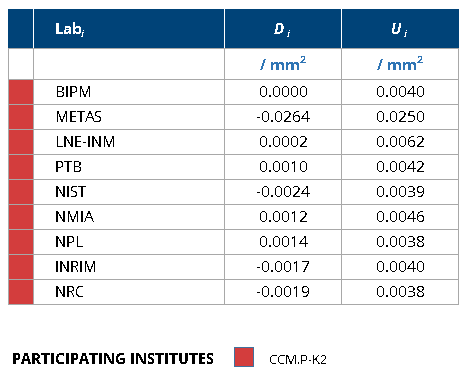CCM.P-K2, EURAMET.M.P-K8 and APMP.M.P-K9

MEASURAND                     Effective area of a piston-cylinder assembly

NOMINAL VALUE              335.7 mm2

NOMINAL PRESSURE       70 kPa

CCM.P-K2 and EURAMET.M.P-K8

Degrees of equivalence represented by Di = (xxR) and its expanded uncertainty Ui at a 95 % level of confidence, both expressed in mm2.

APMP.M.P-K9

Degrees of equivalence represented by Di = (xI - xR) and its expanded uncertainty Ui at a 95 % level of confidence, both expressed in Pa.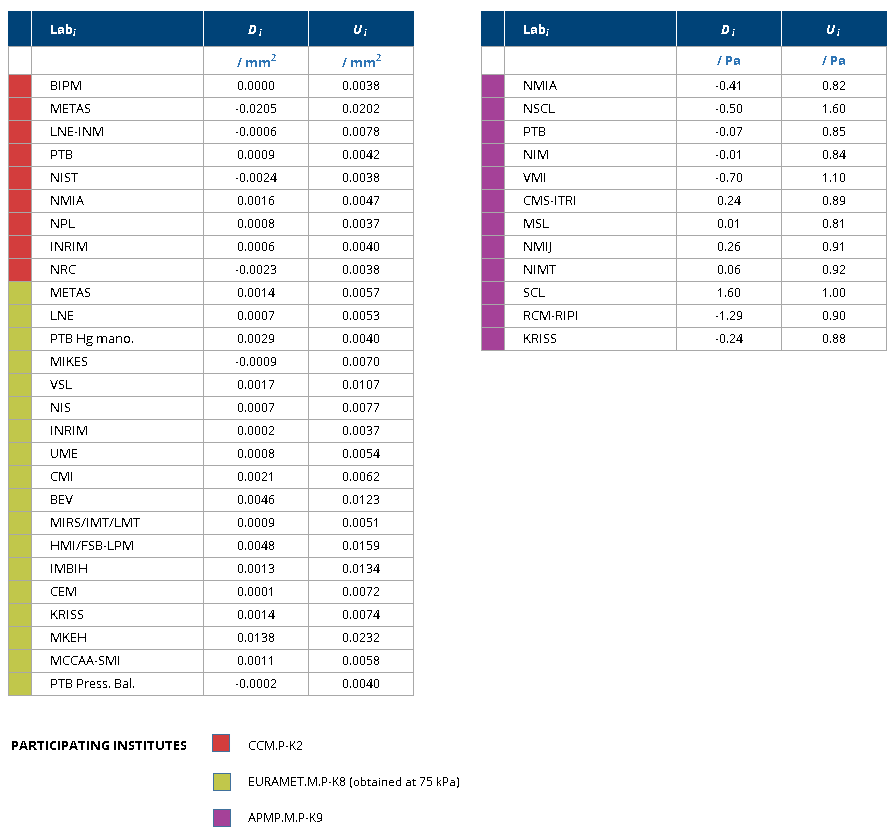CCM.P-K2

MEASURAND                     Effective area of a piston-cylinder assembly

NOMINAL VALUE              335.7 mm2

NOMINAL PRESSURE       80 kPa

Degrees of equivalence represented by Di = (xxR) and its expanded uncertainty Ui at a 95 % level of confidence, both expressed in mm2.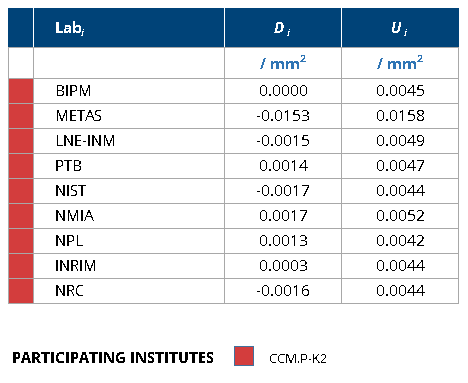CCM.P-K2 and APMP.M.P-K9

MEASURAND                     Effective area of a piston-cylinder assembly

NOMINAL VALUE              335.7 mm2

NOMINAL PRESSURE       90 kPa

CCM.P-K2

Degrees of equivalence represented by Di = (xxR) and its expanded uncertainty Ui at a 95 % level of confidence, both expressed in mm2.

APMP.M.P-K9

Degrees of equivalence represented by Di = (xI - xR) and its expanded uncertainty Ui at a 95 % level of confidence, both expressed in Pa.CCM.P-K2, EURAMET.M.P-K8 and APMP.M.P-K9

MEASURAND                     Effective area of a piston-cylinder assembly

NOMINAL VALUE              335.7 mm2

NOMINAL PRESSURE       100 kPa

CCM.P-K2 and EURAMET.M.P-K8

Degrees of equivalence represented by Di = (xxR) and its expanded uncertainty Ui at a 95 % level of confidence, both expressed in mm2.

APMP.M.P-K9

Degrees of equivalence represented by Di = (xI - xR) and its expanded uncertainty Ui at a 95 % level of confidence, both expressed in Pa.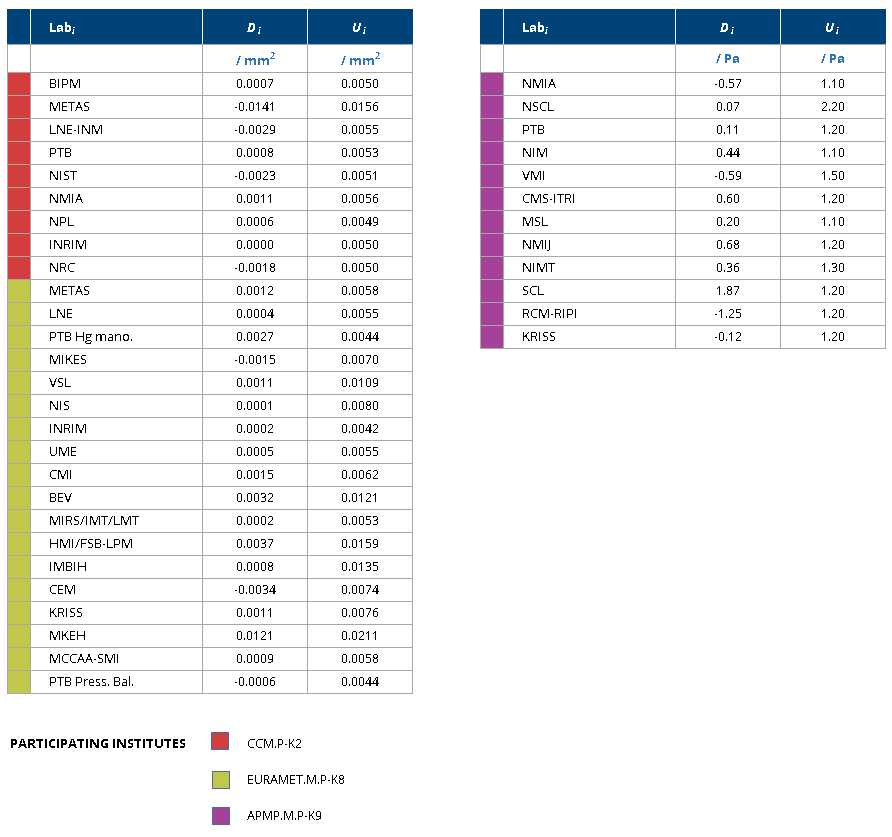CCM.P-K2 and APMP.M.P-K9

MEASURAND                     Effective area of a piston-cylinder assembly

NOMINAL VALUE              335.7 mm2

NOMINAL PRESSURE       110 kPa

CCM.P-K2

Degrees of equivalence represented by Di = (xxR) and its expanded uncertainty Ui at a 95 % level of confidence, both expressed in mm2.

APMP.M.P-K9

Degrees of equivalence represented by Di = (xI - xR) and its expanded uncertainty Ui at a 95 % level of confidence, both expressed in Pa.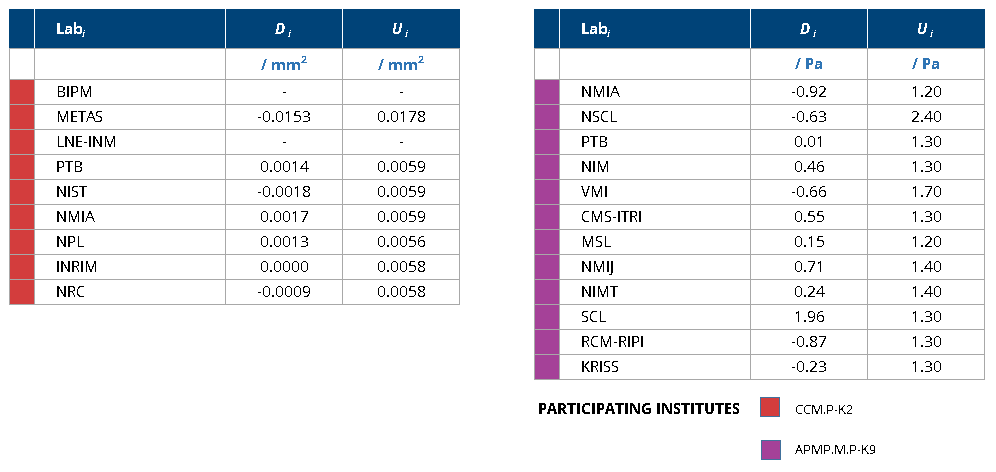CCM.P-K2

MEASURAND                     Effective area of a piston-cylinder assembly

NOMINAL VALUE              335.7 mm2

NOMINAL PRESSURE       120 kPa

Degrees of equivalence represented by Di = (xxR) and its expanded uncertainty Ui at a 95 % level of confidence, both expressed in mm2.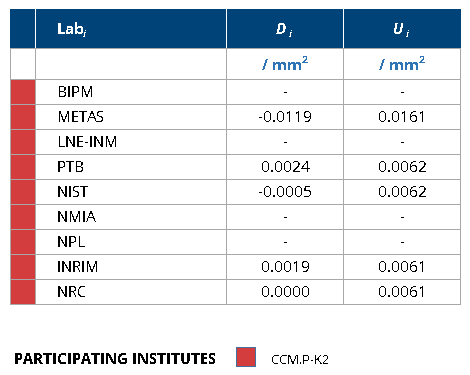Comparison
Comparison close
CC comparison
 Metrology area, Sub-field Mass and related quantities, Pressure Description Pressure meaurements (Absolute mode) Time of measurements 2017 - 2022 Status Measurements in progress
 Measurand Absolute Pressure 10 kPa, 30 kPa, 50 kPa, 70 kPa, 90 kPa, 100 kPa and 110 kPa Transfer device Fluke RPM-4 reference pressure monitor
 Comparison type Supplementary Comparison Consultative Committee CCM (Consultative Committee for Mass and Related Quantities) Conducted by AFRIMETS (Intra-Africa Metrology System) RMO Internal Identifier AFRIMETS.M.P-S1 Comments This supplementary inter laboratory comparison is a replacement of AFRIMETS.M.P-K2 which was abandoned. Pilot institute NMISA National Metrology Institute of South Africa South Africa Contact person Brian YALISI +27728506346
First Name Last Name
wwww@ww.www +356719836 Institute 1 Institute 1 Khmelnitskiy
Pilot laboratory
NMISA

National Metrology Institute of South Africa, South Africa, AFRIMETS

 KEBS Kenya Bureau of Standards, Kenya, AFRIMETS
 LPEE-LNM Laboratoire National de Métrologie du Laboratoire Public d'Essais et d'Études, Morocco, AFRIMETS
 NIS National Institute for Standards, Egypt, AFRIMETS
 PTB Physikalisch-Technische Bundesanstalt, Germany, EURAMET
Comparison
Comparison close
Results

CCM.P-K2, EURAMET.M.P-K4.2010 and APMP.M.P-K9

MEASURAND                     Effective area of a piston-cylinder assembly

NOMINAL VALUE              335.7 mm2

NOMINAL PRESSURE        10 kPa

CCM.P-K2

The key comparison reference value, xR, is calculated as the median of the participants' values. The standard uncertainty of the key comparison reference value, uR, is calculated by the formula uR = 1.858 MAD / (n - 1)1/2, where MAD is the median of absolute deviations from the median, and n is the number of participants contributing to the reference value.

xR = 335.744 4 mm2
uR = 0.000 9 mm2

The degree of equivalence of laboratory i with respect to the key comparison reference value is given by a pair of terms expressed in mm2:

Di = (xi - xR) and Ui = 2(ui2 + uR2 + uT2)1/2, its expanded uncertainty (k = 2). uT is the uncertainty due to the instability of the transfer standard: uT = 0.002 5 mm2 (see page 15 of the Final Report).

The degree of equivalence between two laboratories i and j is given by a pair of terms expressed in mm2Dij = Di - Dj = (xi - xj) and Uij = 2(ui2 + uj2 + 2uT2)1/2, its expanded uncertainty (k = 2). Effects of correlation should be taken into consideration.

PTB provides the link between key comparisons CCM.P-K2 and EURAMET.M.P-K4.2010, having participated in both comparisons. The linkage process is described in Section 11 of the EURAMET.M.P-K4.2010 Final Report.

The degrees of equivalence of the laboratories having participated in EURAMET.M.P-K4.2010, with respect to the CCM.P-K2 key comparison reference value, are expressed in Pa, and therefore are shown on a different graph from the one for the participants in CCM.P-K2.

NMIA and PTB provides the link between key comparisons CCM.P-K2 and APMP.M.P-K9, having participated in both comparisons. The linkage process is described in Section 6 of the APMP.M.P-K9 Final Report.

The degrees of equivalence of the laboratories having participated in APMP.M.P-K9, with respect to the CCM.P-K2 key comparison reference value, are expressed in Pa, and therefore are shown on a different graph from the one for the participants in CCM.P-K2.

CCM.P-K2 and EURAMET.M.P-K8

MEASURAND                     Effective area of a piston-cylinder assembly

NOMINAL VALUE              335.7 mm2

NOMINAL PRESSURE       20 kPa

CCM.P-K2

The key comparison reference value, xR, is calculated as the median of the participants' values. The standard uncertainty of the key comparison reference value, uR, is calculated by the formula uR = 1.858 MAD / (n - 1)1/2, where MAD is the median of absolute deviations from the median, and n is the number of participants contributing to the reference value.

xR = 335.744 8 mm2
uR = 0.001 7 mm2

The degree of equivalence of laboratory i with respect to the key comparison reference value is given by a pair of terms expressed in mm2:

Di = (xi - xR) and Ui = 2(ui2 + uR2 + uT2)1/2, its expanded uncertainty (k = 2). uT is the uncertainty due to the instability of the transfer standard: uT = 0.002 5 mm2 (see page 15 of the Final Report).

The degree of equivalence between two laboratories i and j is given by a pair of terms expressed in mm2Dij = Di - Dj = (xi - xj) and Uij = 2(ui2 + uj2 + 2uT2)1/2, its expanded uncertainty (k = 2). Effects of correlation should be taken into consideration.

PTB and INRiM provides the link between key comparisons CCM.P-K2 and EURAMET.M.P-K8, having participated in both comparisons. The linkage process is described in Section 11 of the EURAMET.M.P-K8 Final Report.

The degrees of equivalence of the laboratories having participated in EURAMET.M.P-K4.2010, with respect to the CCM.P-K2 key comparison reference value, are expressed in Pa, and therefore are shown on a different graph from the one for the participants in CCM.P-K2.

CCM.P-K2 and APMP.M.P-K9

MEASURAND                     Effective area of a piston-cylinder assembly

NOMINAL VALUE              335.7 mm2

NOMINAL PRESSURE       30 kPa

CCM.P-K2

The key comparison reference value, xR, is calculated as the median of the participants' values. The standard uncertainty of the key comparison reference value, uR, is calculated by the formula uR = 1.858 MAD / (n - 1)1/2, where MAD is the median of absolute deviations from the median, and n is the number of participants contributing to the reference value.

xR = 335.745 5 mm2
uR = 0.001 0 mm2

The degree of equivalence of laboratory i with respect to the key comparison reference value is given by a pair of terms expressed in mm2:

Di = (xi - xR) and Ui = 2(ui2 + uR2 + uT2)1/2, its expanded uncertainty (k = 2). uT is the uncertainty due to the instability of the transfer standard: uT = 0.002 5 mm2 (see page 15 of the Final Report).

The degree of equivalence between two laboratories i and j is given by a pair of terms expressed in mm2Dij = Di - Dj = (xi - xj) and Uij = 2(ui2 + uj2 + 2uT2)1/2, its expanded uncertainty (k = 2). Effects of correlation should be taken into consideration.

NMIA and PTB provides the link between key comparisons CCM.P-K2 and APMP.M.P-K9, having participated in both comparisons. The linkage process is described in Section 6 of the APMP.M.P-K9 Final Report.

The degrees of equivalence of the laboratories having participated in APMP.M.P-K9, with respect to the CCM.P-K2 key comparison reference value, are expressed in Pa, and therefore are shown on a different graph from the one for the participants in CCM.P-K2.

CCM.P-K2

MEASURAND                     Effective area of a piston-cylinder assembly

NOMINAL VALUE              335.7 mm2

NOMINAL PRESSURE       40 kPa

The key comparison reference value, xR, is calculated as the median of the participants' values. The standard uncertainty of the key comparison reference value, uR, is calculated by the formula uR = 1.858 MAD / (n - 1)1/2, where MAD is the median of absolute deviations from the median, and n is the number of participants contributing to the reference value.

xR = 335.744 2 mm2
uR = 0.000 8 mm2

The degree of equivalence of laboratory i with respect to the key comparison reference value is given by a pair of terms expressed in mm2:

Di = (xi - xR) and Ui = 2(ui2 + uR2 + uT2)1/2, its expanded uncertainty (k = 2). uT is the uncertainty due to the instability of the transfer standard: uT = 0.002 5 mm2 (see page 15 of the Final Report).

The degree of equivalence between two laboratories i and j is given by a pair of terms expressed in mm2Dij = Di - Dj = (xi - xj) and Uij = 2(ui2 + uj2 + 2uT2)1/2, its expanded uncertainty (k = 2). Effects of correlation should be taken into consideration.

CCM.P-K2, EURAMET.M.P-K8 and APMP.M.P-K9

MEASURAND                     Effective area of a piston-cylinder assembly

NOMINAL VALUE              335.7 mm2

NOMINAL PRESSURE       50 kPa

CCM.P-K2

The key comparison reference value, xR, is calculated as the median of the participants' values. The standard uncertainty of the key comparison reference value, uR, is calculated by the formula uR = 1.858 MAD / (n - 1)1/2, where MAD is the median of absolute deviations from the median, and n is the number of participants contributing to the reference value.

xR = 335.744 0 mm2
uR = 0.000 8 mm2

The degree of equivalence of laboratory i with respect to the key comparison reference value is given by a pair of terms expressed in mm2:

Di = (xi - xR) and Ui = 2(ui2 + uR2 + uT2)1/2, its expanded uncertainty (k = 2). uT is the uncertainty due to the instability of the transfer standard: uT = 0.002 5 mm2 (see page 15 of the Final Report).

The degree of equivalence between two laboratories i and j is given by a pair of terms expressed in mm2Dij = Di - Dj = (xi - xj) and Uij = 2(ui2 + uj2 + 2uT2)1/2, its expanded uncertainty (k = 2). Effects of correlation should be taken into consideration.

PTB and INRiM provides the link between key comparisons CCM.P-K2 and EURAMET.M.P-K8, having participated in both comparisons. The linkage process is described in Section 11 of the EURAMET.M.P-K8 Final Report.

The degrees of equivalence of the laboratories having participated in EURAMET.M.P-K4.2010, with respect to the CCM.P-K2 key comparison reference value, are expressed in Pa, and therefore are shown on a different graph from the one for the participants in CCM.P-K2.

NMIA and PTB provides the link between key comparisons CCM.P-K2 and APMP.M.P-K9, having participated in both comparisons. The linkage process is described in Section 6 of the APMP.M.P-K9 Final Report.

The degrees of equivalence of the laboratories having participated in APMP.M.P-K9, with respect to the CCM.P-K2 key comparison reference value, are expressed in Pa, and therefore are shown on a different graph from the one for the participants in CCM.P-K2.

CCM.P-K2

MEASURAND                     Effective area of a piston-cylinder assembly

NOMINAL VALUE              335.7 mm2

NOMINAL PRESSURE       60 kPa

The key comparison reference value, xR, is calculated as the median of the participants' values. The standard uncertainty of the key comparison reference value, uR, is calculated by the formula uR = 1.858 MAD / (n - 1)1/2, where MAD is the median of absolute deviations from the median, and n is the number of participants contributing to the reference value.

xR = 335.745 1 mm2
uR = 0.000 9 mm2

The degree of equivalence of laboratory i with respect to the key comparison reference value is given by a pair of terms expressed in mm2:

Di = (xi - xR) and Ui = 2(ui2 + uR2 + uT2)1/2, its expanded uncertainty (k = 2). uT is the uncertainty due to the instability of the transfer standard: uT = 0.002 5 mm2 (see page 15 of the Final Report).

The degree of equivalence between two laboratories i and j is given by a pair of terms expressed in mm2Dij = Di - Dj = (xi - xj) and Uij = 2(ui2 + uj2 + 2uT2)1/2, its expanded uncertainty (k = 2). Effects of correlation should be taken into consideration.

CCM.P-K2, EURAMET.M.P-K8 and APMP.M.P-K9

MEASURAND                     Effective area of a piston-cylinder assembly

NOMINAL VALUE              335.7 mm2

NOMINAL PRESSURE       70 kPa

CCM.P-K2

The key comparison reference value, xR, is calculated as the median of the participants' values. The standard uncertainty of the key comparison reference value, uR, is calculated by the formula uR = 1.858 MAD / (n - 1)1/2, where MAD is the median of absolute deviations from the median, and n is the number of participants contributing to the reference value.

xR = 335.745 3 mm2
uR = 0.000 5 mm2

The degree of equivalence of laboratory i with respect to the key comparison reference value is given by a pair of terms expressed in mm2:

Di = (xi - xR) and Ui = 2(ui2 + uR2 + uT2)1/2, its expanded uncertainty (k = 2). uT is the uncertainty due to the instability of the transfer standard: uT = 0.002 5 mm2 (see page 15 of the Final Report).

The degree of equivalence between two laboratories i and j is given by a pair of terms expressed in mm2Dij = Di - Dj = (xi - xj) and Uij = 2(ui2 + uj2 + 2uT2)1/2, its expanded uncertainty (k = 2). Effects of correlation should be taken into consideration.

PTB and INRiM provides the link between key comparisons CCM.P-K2 and EURAMET.M.P-K8, having participated in both comparisons. The linkage process is described in Section 11 of the EURAMET.M.P-K8 Final Report.

The degrees of equivalence of the laboratories having participated in EURAMET.M.P-K4.2010, with respect to the CCM.P-K2 key comparison reference value, are expressed in Pa, and therefore are shown on a different graph from the one for the participants in CCM.P-K2.

NMIA and PTB provides the link between key comparisons CCM.P-K2 and APMP.M.P-K9, having participated in both comparisons. The linkage process is described in Section 6 of the APMP.M.P-K9 Final Report.

The degrees of equivalence of the laboratories having participated in APMP.M.P-K9, with respect to the CCM.P-K2 key comparison reference value, are expressed in Pa, and therefore are shown on a different graph from the one for the participants in CCM.P-K2.

CCM.P-K2

MEASURAND                     Effective area of a piston-cylinder assembly

NOMINAL VALUE              335.7 mm2

NOMINAL PRESSURE       80 kPa

The key comparison reference value, xR, is calculated as the median of the participants' values. The standard uncertainty of the key comparison reference value, uR, is calculated by the formula uR = 1.858 MAD / (n - 1)1/2, where MAD is the median of absolute deviations from the median, and n is the number of participants contributing to the reference value.

xR = 335.744 6 mm2
uR = 0.000 9 mm2

The degree of equivalence of laboratory i with respect to the key comparison reference value is given by a pair of terms expressed in mm2:

Di = (xi - xR) and Ui = 2(ui2 + uR2 + uT2)1/2, its expanded uncertainty (k = 2). uT is the uncertainty due to the instability of the transfer standard: uT = 0.002 5 mm2 (see page 15 of the Final Report).

The degree of equivalence between two laboratories i and j is given by a pair of terms expressed in mm2Dij = Di - Dj = (xi - xj) and Uij = 2(ui2 + uj2 + 2uT2)1/2, its expanded uncertainty (k = 2). Effects of correlation should be taken into consideration.

CCM.P-K2 and APMP.M.P-K9

MEASURAND                     Effective area of a piston-cylinder assembly

NOMINAL VALUE              335.7 mm2

NOMINAL PRESSURE       90 kPa

CCM.P-K2

CCM.P-K2

The key comparison reference value, xR, is calculated as the median of the participants' values. The standard uncertainty of the key comparison reference value, uR, is calculated by the formula uR = 1.858 MAD / (n - 1)1/2, where MAD is the median of absolute deviations from the median, and n is the number of participants contributing to the reference value.

xR = 335.744 8 mm2
uR = 0.000 9 mm2

The degree of equivalence of laboratory i with respect to the key comparison reference value is given by a pair of terms expressed in mm2:

Di = (xi - xR) and Ui = 2(ui2 + uR2 + uT2)1/2, its expanded uncertainty (k = 2). uT is the uncertainty due to the instability of the transfer standard: uT = 0.002 5 mm2 (see page 15 of the Final Report).

The degree of equivalence between two laboratories i and j is given by a pair of terms expressed in mm2Dij = Di - Dj = (xi - xj) and Uij = 2(ui2 + uj2 + 2uT2)1/2, its expanded uncertainty (k = 2). Effects of correlation should be taken into consideration.

NMIA and PTB provides the link between key comparisons CCM.P-K2 and APMP.M.P-K9, having participated in both comparisons. The linkage process is described in Section 6 of the APMP.M.P-K9 Final Report.

The degrees of equivalence of the laboratories having participated in APMP.M.P-K9, with respect to the CCM.P-K2 key comparison reference value, are expressed in Pa, and therefore are shown on a different graph from the one for the participants in CCM.P-K2.

CCM.P-K2, EURAMET.M.P-K8 and APMP.M.P-K9

MEASURAND                     Effective area of a piston-cylinder assembly

NOMINAL VALUE              335.7 mm2

NOMINAL PRESSURE       100 kPa

CCM.P-K2

The key comparison reference value, xR, is calculated as the median of the participants' values. The standard uncertainty of the key comparison reference value, uR, is calculated by the formula uR = 1.858 MAD / (n - 1)1/2, where MAD is the median of absolute deviations from the median, and n is the number of participants contributing to the reference value.

xR = 335.745 1 mm2
uR = 0.000 7 mm2

The degree of equivalence of laboratory i with respect to the key comparison reference value is given by a pair of terms expressed in mm2:

Di = (xi - xR) and Ui = 2(ui2 + uR2 + uT2)1/2, its expanded uncertainty (k = 2). uT is the uncertainty due to the instability of the transfer standard: uT = 0.002 5 mm2 (see page 15 of the Final Report).

The degree of equivalence between two laboratories i and j is given by a pair of terms expressed in mm2Dij = Di - Dj = (xi - xj) and Uij = 2(ui2 + uj2 + 2uT2)1/2, its expanded uncertainty (k = 2). Effects of correlation should be taken into consideration.

PTB and INRiM provides the link between key comparisons CCM.P-K2 and EURAMET.M.P-K8, having participated in both comparisons. The linkage process is described in Section 11 of the EURAMET.M.P-K8 Final Report.

The degrees of equivalence of the laboratories having participated in EURAMET.M.P-K4.2010, with respect to the CCM.P-K2 key comparison reference value, are expressed in Pa, and therefore are shown on a different graph from the one for the participants in CCM.P-K2.

NMIA and PTB provides the link between key comparisons CCM.P-K2 and APMP.M.P-K9, having participated in both comparisons. The linkage process is described in Section 6 of the APMP.M.P-K9 Final Report.

The degrees of equivalence of the laboratories having participated in APMP.M.P-K9, with respect to the CCM.P-K2 key comparison reference value, are expressed in Pa, and therefore are shown on a different graph from the one for the participants in CCM.P-K2.

CCM.P-K2 and APMP.M.P-K9

MEASURAND                     Effective area of a piston-cylinder assembly

NOMINAL VALUE              335.7 mm2

NOMINAL PRESSURE       110 kPa

CCM.P-K2

The key comparison reference value, xR, is calculated as the median of the participants' values. The standard uncertainty of the key comparison reference value, uR, is calculated by the formula uR = 1.858 MAD / (n - 1)1/2, where MAD is the median of absolute deviations from the median, and n is the number of participants contributing to the reference value.

xR = 335.744 3 mm2
uR = 0.001 0 mm2

The degree of equivalence of laboratory i with respect to the key comparison reference value is given by a pair of terms expressed in mm2:

Di = (xi - xR) and Ui = 2(ui2 + uR2 + uT2)1/2, its expanded uncertainty (k = 2). uT is the uncertainty due to the instability of the transfer standard: uT = 0.002 5 mm2 (see page 15 of the Final Report).

The degree of equivalence between two laboratories i and j is given by a pair of terms expressed in mm2Dij = Di - Dj = (xi - xj) and Uij = 2(ui2 + uj2 + 2uT2)1/2, its expanded uncertainty (k = 2). Effects of correlation should be taken into consideration.

NMIA and PTB provides the link between key comparisons CCM.P-K2 and APMP.M.P-K9, having participated in both comparisons. The linkage process is described in Section 6 of the APMP.M.P-K9 Final Report.

The degrees of equivalence of the laboratories having participated in APMP.M.P-K9, with respect to the CCM.P-K2 key comparison reference value, are expressed in Pa, and therefore are shown on a different graph from the one for the participants in CCM.P-K2.

CCM.P-K2

MEASURAND                     Effective area of a piston-cylinder assembly

NOMINAL VALUE              335.7 mm2

NOMINAL PRESSURE       120 kPa

The key comparison reference value, xR, is calculated as the median of the participants' values. The standard uncertainty of the key comparison reference value, uR, is calculated by the formula uR = 1.858 MAD / (n - 1)1/2, where MAD is the median of absolute deviations from the median, and n is the number of participants contributing to the reference value.

xR = 335.743 2 mm2
uR = 0.001 6 mm2

The degree of equivalence of laboratory i with respect to the key comparison reference value is given by a pair of terms expressed in mm2:

Di = (xi - xR) and Ui = 2(ui2 + uR2 + uT2)1/2, its expanded uncertainty (k = 2). uT is the uncertainty due to the instability of the transfer standard: uT = 0.002 5 mm2 (see page 15 of the Final Report).

The degree of equivalence between two laboratories i and j is given by a pair of terms expressed in mm2Dij = Di - Dj = (xi - xj) and Uij = 2(ui2 + uj2 + 2uT2)1/2, its expanded uncertainty (k = 2). Effects of correlation should be taken into consideration.

Comparison
Comparison close
Results

CCM.P-K2, EURAMET.M.P-K4.2010 and APMP.M.P-K9

MEASURAND                     Effective area of a piston-cylinder assembly

NOMINAL VALUE              335.7 mm2

NOMINAL PRESSURE        10 kPa

xi   results obtained by laboratory i, Labi

ui   combined standard uncertainty of xi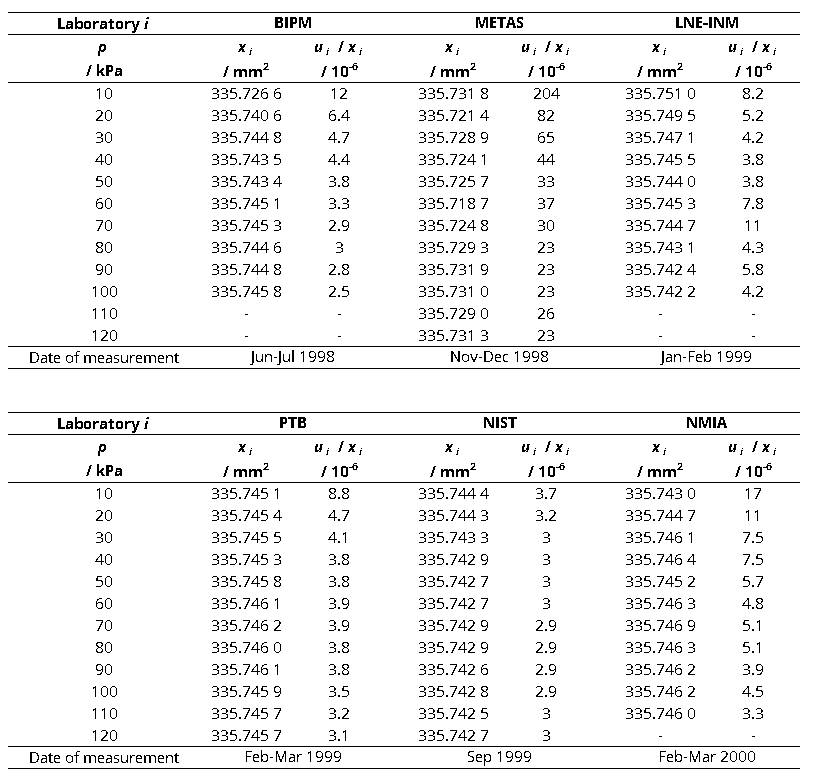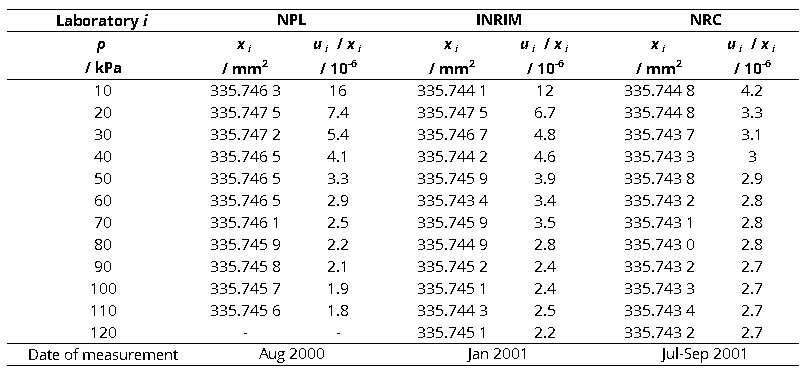CCM.P-K2 and EURAMET.M.P-K8

MEASURAND                     Effective area of a piston-cylinder assembly

NOMINAL VALUE              335.7 mm2

NOMINAL PRESSURE       20 kPa

xi   results obtained by laboratory i, Labi

ui   combined standard uncertainty of xiCCM.P-K2 and APMP.M.P-K9

MEASURAND                     Effective area of a piston-cylinder assembly

NOMINAL VALUE              335.7 mm2

NOMINAL PRESSURE       30 kPa

xi   results obtained by laboratory i, Labi

ui   combined standard uncertainty of xiCCM.P-K2

MEASURAND                     Effective area of a piston-cylinder assembly

NOMINAL VALUE              335.7 mm2

NOMINAL PRESSURE       40 kPa

xi   results obtained by laboratory i, Labi

ui   combined standard uncertainty of xiCCM.P-K2, EURAMET.M.P-K8 and APMP.M.P-K9

MEASURAND                     Effective area of a piston-cylinder assembly

NOMINAL VALUE              335.7 mm2

NOMINAL PRESSURE       50 kPa

xi   results obtained by laboratory i, Labi

ui   combined standard uncertainty of xiCCM.P-K2

MEASURAND                     Effective area of a piston-cylinder assembly

NOMINAL VALUE              335.7 mm2

NOMINAL PRESSURE       60 kPa

xi   results obtained by laboratory i, Labi

ui   combined standard uncertainty of xiCCM.P-K2, EURAMET.M.P-K8 and APMP.M.P-K9

MEASURAND                     Effective area of a piston-cylinder assembly

NOMINAL VALUE              335.7 mm2

NOMINAL PRESSURE       70 kPa

xi   results obtained by laboratory i, Labi

ui   combined standard uncertainty of xiCCM.P-K2

MEASURAND                     Effective area of a piston-cylinder assembly

NOMINAL VALUE              335.7 mm2

NOMINAL PRESSURE       80 kPa

xi   results obtained by laboratory i, Labi

ui   combined standard uncertainty of xiCCM.P-K2 and APMP.M.P-K9

MEASURAND                     Effective area of a piston-cylinder assembly

NOMINAL VALUE              335.7 mm2

NOMINAL PRESSURE       90 kPa

xi   results obtained by laboratory i, Labi

ui   combined standard uncertainty of xiCCM.P-K2, EURAMET.M.P-K8 and APMP.M.P-K9

MEASURAND                     Effective area of a piston-cylinder assembly

NOMINAL VALUE              335.7 mm2

NOMINAL PRESSURE       100 kPa

xi   results obtained by laboratory i, Labi

ui   combined standard uncertainty of xiCCM.P-K2 and APMP.M.P-K9

MEASURAND                     Effective area of a piston-cylinder assembly

NOMINAL VALUE              335.7 mm2

NOMINAL PRESSURE       110 kPa

xi   results obtained by laboratory i, Labi

ui   combined standard uncertainty of xiCCM.P-K2

MEASURAND                     Effective area of a piston-cylinder assembly

NOMINAL VALUE              335.7 mm2

NOMINAL PRESSURE       120 kPa

xi   results obtained by laboratory i, Labi

ui   combined standard uncertainty of xi